## Spam Detection

Feb 9, 2019| Category: Security|

Spams is a cost-effective method for advertisement. Only a very small fraction of the recipients may get interested in the product, but this is a big problem for most users. Spammers collect recipients contact from publicly accessible sources and make use of this medium to advertise their products. At present more than 95% of the emails sent are believed to be spam and this makes spams a severe problem.

To avoid this, is it possible to detect Spam using machine learning?
Let us look at a dataset of spam sms by UCI 1 and see if we can use the power of machine learning to detect spams!

#### 4) Summary

In :
from IPython.display import display
from IPython.display import HTML
import IPython.core.display as di # Example: di.display_html('<h3>%s:</h3>' % str, raw=True)

# This line will hide code by default when the notebook is exported as HTML
di.display_html('<script>jQuery(function() {if (jQuery("body.notebook_app").length == 0) { jQuery(".input_area").toggle(); jQuery(".prompt").toggle();}});</script>', raw=True)

# This line will add a button to toggle visibility of code blocks, for use with the HTML export version
di.display_html('''<button onclick="jQuery('.input_area').toggle(); jQuery('.prompt').toggle();">Toggle code</button>''', raw=True)

In :
#Importing the required libraries
import numpy as np
import pandas as pd
import seaborn as sns
import matplotlib.pyplot as plt
from sklearn import feature_extraction
from sklearn.naive_bayes import MultinomialNB
from sklearn.model_selection import GridSearchCV
from sklearn.base import BaseEstimator, TransformerMixin
from sklearn.pipeline import Pipeline
from sklearn import metrics

import warnings
warnings.filterwarnings("ignore")
%matplotlib inline

from collections import Counter
from nltk.tokenize import RegexpTokenizer
from stop_words import get_stop_words
import nltk
from nltk.corpus import stopwords
from nltk import sent_tokenize, word_tokenize

In :
#We will read the spam sms datasetand perform some EDA
df = df.iloc[:,0:2]
df.columns = ['class' , 'Text']


### Descriptive Statistics¶

We have a sms dataset containing spams and ham. The goal is to predict whether the text is a spam or not using machine learning. We will make use of Natural language processing techniques train a model and perform spam detection.

In :
df.head()

Out:
Sms dataset
class Text
0 ham Go until jurong point, crazy.. Available only ...
1 ham Ok lar... Joking wif u oni...
2 spam Free entry in 2 a wkly comp to win FA Cup fina...
3 ham U dun say so early hor... U c already then say...
4 ham Nah I don't think he goes to usf, he lives aro...

The dataset consist of text message along with the information whether it is a spam or not.

### Exploratory data analysis¶

In :
sns.countplot(df['class'])
plt.show()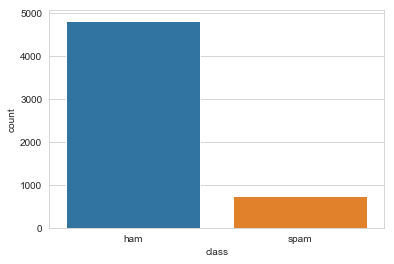We can see most of the sms are genuine messages while some are spams. Let us look if there is a relationship between the length of a message and the type of message.

In :
df['Text']=df['Text'].astype(str)
df['Text length']=df['Text'].apply(len)
sns.barplot(x= 'class', y = 'Text length', data = df)
plt.show()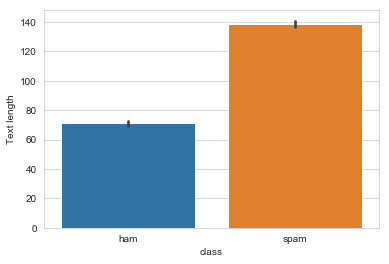Spam messages are longer than genuine messages on average, interesting!Let us visualize this with a boxplot.

In :
sns.boxplot(x= 'class', y = 'Text length', data = df)
plt.show()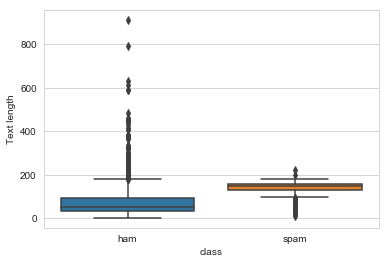On average genuine messages are shorter than spams, but we can see it is not always the case as we can see a lot of outliers for ham.
Let us find the most frequetly used words in spam and ham messages

In :
data_spam = df[df['class'] == 'spam']
data_ham = df[df['class'] == 'ham']
#Seperating all the words to create a bag of words model
spam = data_spam['Text'].str.lower().str.cat(sep=' ')
ham =  data_ham['Text'].str.lower().str.cat(sep=' ')


Let us clean the data first by removing stop words. Stop words usually refers to the most common words in a language which are mostly grammar words that wouldn't be useful for this text analysis. Let us have a look at the most common stop words.

In :
stop_words = list(get_stop_words('en'))
nltk_words = list(stopwords.words('english'))
stop_words.extend(nltk_words)
print(stop_words[0:30])

['a', 'about', 'above', 'after', 'again', 'against', 'all', 'am', 'an', 'and', 'any', 'are', "aren't", 'as', 'at', 'be', 'because', 'been', 'before', 'being', 'below', 'between', 'both', 'but', 'by', "can't", 'cannot', 'could', "couldn't", 'did']


These words are unnecessary for the analysis and we just eliminate them.

In :
#A function to remove the stop words, numeric data from the bag of words model
def top_words(a):
word_tokens = word_tokenize(a)
filtered_sentence = []
for w in word_tokens:
if w not in stop_words:
filtered_sentence.append(w)
len(filtered_sentence)

# Remove characters which have length less than 2
without_single_chr = [word for word in filtered_sentence if len(word) > 2]

# Remove numbers
global cleaned_data_title
cleaned_data_title = [word for word in without_single_chr if not word.isnumeric()]
top_N = 100
word_dist = nltk.FreqDist(cleaned_data_title)
rslt = pd.DataFrame(word_dist.most_common(top_N),columns=['Word', 'Frequency'])
plt.figure(figsize=(14,5))
sns.set_style("whitegrid")


Let us look at the top 15 words that are present is a genuine message

In :
top_words(ham)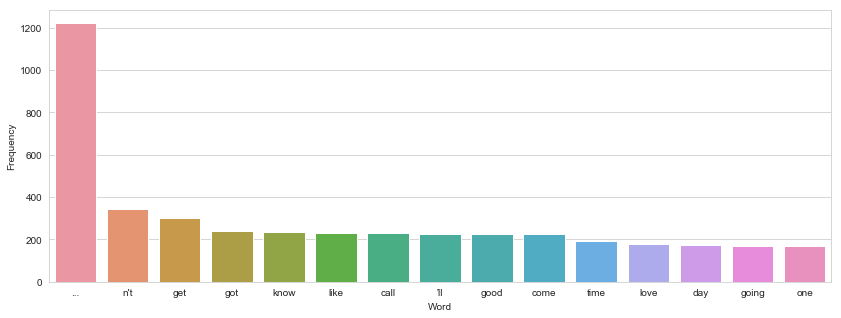We can see that genuine messages have commonly used words in a normal text message. The most common word is .. which are called ellipsis indicating a pause or silence and is very commonly used in texting. Other words in the bar plot are words that are commonly used in a normal conversation.

In :
top_words(spam)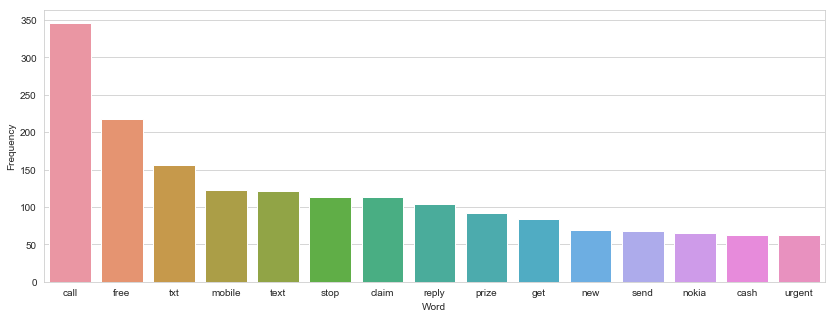We can see the most common words are call, free, cash, prize or winning and is so different from the bar plot we saw for genuine messages. Maybe these differences are detected by machine learning models to distinguish between a spam and a genuine message.

Let us visualize the most commonly used words for a spam message in a word cloud.

In :
from wordcloud import WordCloud, STOPWORDS
def wc(data,bgcolor,title):
plt.figure(figsize = (60,60))
wc = WordCloud(background_color = bgcolor, max_words = 1000,  max_font_size = 100)
wc.generate(' '.join(data))
plt.imshow(wc)
plt.axis('off')

wc(cleaned_data_title,'white','Most Used Words')### Machine Learning¶

Let us make use of machine learning to predict whether a message is a spam or ham

In :
#Converting into numerical values so that it can be interpreted by the machine.
df["class"]=df["class"].map({'spam':1,'ham':0})

#Diving the data into training and testing datasets.
train=df.sample(frac=0.75,random_state=200)
test=df.drop(train.index)


While creating a model we need to make sure that it is good in detecting spams but more importantly the model should never classify a genuine message as a spam. It means it should should have a low false positive rate!

If a spam is classied as normal message is fine but it should be the other way around! An important message should never go to the spam folder.

We will make use of pipelining and gridsearch in order to take advantage of cross validation and train a model with the focus on precision so that there is a low false positive rate.

In :
#Let us create a class for preprocessing which will be used as a step for the pipline.
class PreProcessing(BaseEstimator, TransformerMixin):
"""Custom Pre-Processing estimator for our use-case
"""

def __init__(self):
pass

def transform(self, df):
df = df.astype(str)
return df

def fit(self, df, y=None, **fit_params):
return self

#Let us try Naive Bayes which workds great with text data.
clf = MultinomialNB()

#This is our pipeline with Preprocessing, countvectorizer steps
pipe = Pipeline([('pre', PreProcessing()),('cv', feature_extraction.text.CountVectorizer()), ('clf', clf)])

#We will make use of grid search to select optimum parameter alpha for Naive bayes by making use of cross validation.
params = {'clf__alpha':np.arange(1,30,3)}
grid = GridSearchCV(pipe,params, cv = 3, scoring='accuracy')

grid.fit(train['Text'], train['class'])

Out:
GridSearchCV(cv=3, error_score='raise-deprecating',
estimator=Pipeline(memory=None,
steps=[('pre', PreProcessing()), ('cv', CountVectorizer(analyzer='word', binary=False, decode_error='strict',
dtype=<class 'numpy.int64'>, encoding='utf-8', input='content',
lowercase=True, max_df=1.0, max_features=None, min_df=1,
ngram_ra...nizer=None, vocabulary=None)), ('clf', MultinomialNB(alpha=1.0, class_prior=None, fit_prior=True))]),
fit_params=None, iid='warn', n_jobs=None,
param_grid={'clf__alpha': array([ 1,  4,  7, 10, 13, 16, 19, 22, 25, 28])},
pre_dispatch='2*n_jobs', refit=True, return_train_score='warn',
scoring='accuracy', verbose=0)

Let us look at the predictions on the Test dataset.

In :
predictions = grid.predict(test['Text'])
test['prediction'] = predictions

Out:
class Text Text length prediction
2 1 Free entry in 2 a wkly comp to win FA Cup fina... 155 1
17 0 Eh u remember how 2 spell his name... Yes i di... 81 0
18 0 Fine if thatåÕs the way u feel. ThatåÕs the wa... 58 0
37 0 I see the letter B on my car 28 0
40 0 Pls go ahead with watts. I just wanted to be s... 82 0
46 0 Didn't you get hep b immunisation in nigeria. 45 0
48 0 Yeah hopefully, if tyler can't do it I could m... 67 0
57 0 Sorry, I'll call later in meeting. 34 0
61 0 Ha ha ha good joke. Girls are situation seekers. 48 0
65 1 As a valued customer, I am pleased to advise y... 153 1

Let us calculate the accuracy of our model

In :
m_confusion_test = metrics.confusion_matrix(test['class'], test['prediction'])
pd.DataFrame(data = m_confusion_test, columns = ['Predicted Not spam', 'Predicted Spam'],
index = ['Actual not spam', 'Actual spam'])

Out:
Confusion matrix
Predicted Not spam Predicted Spam
Actual not spam 1194 4
Actual spam 16 179
In :
print('Hence we have achieved {}% accuracy in detecting whether a message is a spam or not.' .format(round(1373/1393 * 100,2)))

Hence we have achieved 98.56% accuracy in detecting whether a message is a spam or not.


### Summary¶

Thus we have performed analysis on the UCI sms dataset. We can see how machine interprets text data and create a classier based on how we train a model.

We also saw how important is cleaning and the use of countvectorizer to convert a collection of textual data into a matrix of token counts. This is important as a machine would not understand the meaning of a word. But if it classifies the word according to the frequency it can create a mathematical model that has the same meaning as that of the text document.

Also we saw that spam detections should should have a low false positive rate, that means if a spam is classied as a normal message it is bad but its worse if its the other way around! An important message should never go to the spam folder.

1. Data Source: https://archive.ics.uci.edu/ml/datasets/sms+spam+collection